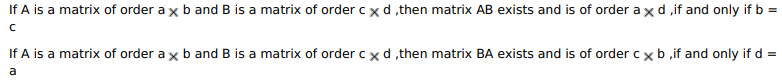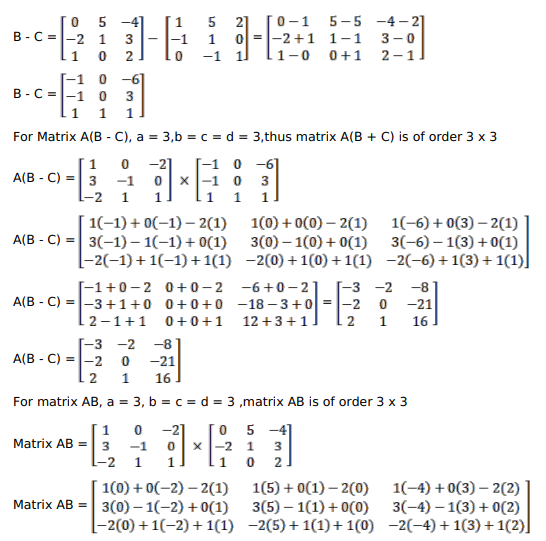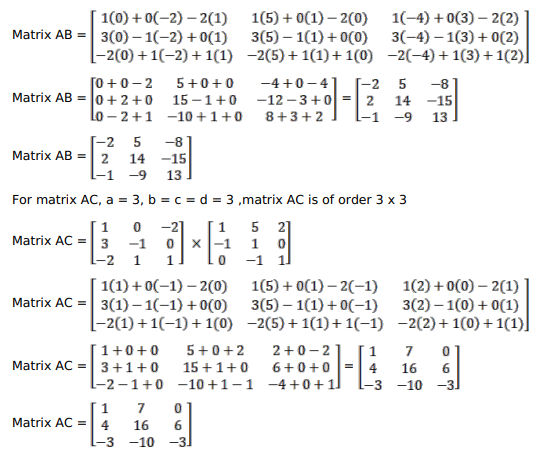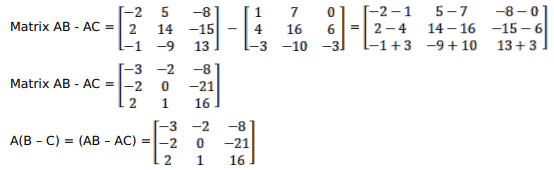Deepak Scored 45->99%ile with Bounce Back Crack Course. You can do it too!

# Solve this following

Question:

If $\mathrm{A}=\left[\begin{array}{ccc}1 & 0 & -2 \\ 3 & -1 & 0 \\ -2 & 1 & 1\end{array}\right], B=\left[\begin{array}{ccc}0 & 5 & -4 \\ -2 & 1 & 3 \\ 1 & 0 & 2\end{array}\right]$ and $C=\left[\begin{array}{ccc}1 & 5 & 2 \\ -1 & 1 & 0 \\ 0 & -1 & 1\end{array}\right]$; verify that $A(B-C)=(A B-A C)$

Solution: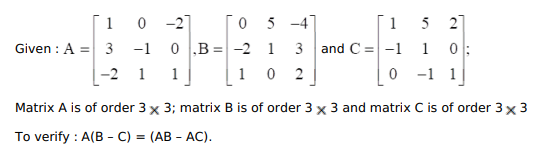$\left[\begin{array}{lll}a_{11} & a_{12} & a_{13} \\ a_{21} & a_{22} & a_{23} \\ a_{31} & a_{32} & a_{33}\end{array}\right] \times\left[\begin{array}{lll}b_{11} & b_{12} & b_{13} \\ b_{21} & b_{22} & b_{23} \\ b_{31} & b_{32} & b_{33}\end{array}\right]$

$=\left[\begin{array}{lll}a_{11} b_{11}+a_{12} b_{21}+a_{13} b_{31} & a_{11} b_{12}+a_{12} b_{22}+a_{13} b_{32} & a_{11} b_{13}+a_{12} b_{23}+a_{13} b_{33} \\ a_{21} b_{11}+a_{22} b_{21}+a_{23} b_{31} & a_{21} b_{12}+a_{22} b_{22}+a_{23} b_{32} & a_{21} b_{13}+a_{22} b_{23}+a_{23} b_{33} \\ a_{31} b_{11}+a_{32} b_{21}+a_{33} b_{31} & a_{31} b_{12}+a_{32} b_{22}+a_{33} b_{32} & a_{31} b_{13}+a_{32} b_{23}+a_{33} b_{33}\end{array}\right]$